﻿ Theoretical Basics of Geometric Mechanics and Differential Geometry

### Theoretical Basics of Geometric Mechanics and Differential Geometry

Tomáš Lipták, Alexander Gmiterko, Michal Kelemen, Ivan Virgala, Erik Prada, František MendaOPEN ACCESSPEER-REVIEWED

## Theoretical Basics of Geometric Mechanics and Differential Geometry

Tomáš Lipták1,, Alexander Gmiterko1, Michal Kelemen1, Ivan Virgala1, Erik Prada1, František Menda2

1Department of Mechatronics, Faculty of Mechanical Engineering, Technical University of Košice, Košice, Slovakia

2Department of Applied mechanics and mechanical engineering, Faculty of Mechanical Engineering, Technical University of Košice, Košice, Slovakia

### Abstract

In this article are described the theoretical basics of geometric mechanics and differential geometry. In the introductory part of article, basic notions are explained that frequently occurring in the concept of geometric mechanics. It contains the basic building blocks that are used to create the configuration spaces. Further were described groups applied to the kinematic description of structure and to control in area of robotics and the fiber bundle that represents the configuration space for mechanical systems. The last part deals with the actions, such as the left and right action, lifted action and adjoint action.

### At a glance: Figures

1234
Prev Next

• Lipták, Tomáš, et al. "Theoretical Basics of Geometric Mechanics and Differential Geometry." American Journal of Mechanical Engineering 2.7 (2014): 178-183.
• Lipták, T. , Gmiterko, A. , Kelemen, M. , Virgala, I. , Prada, E. , & Menda, F. (2014). Theoretical Basics of Geometric Mechanics and Differential Geometry. American Journal of Mechanical Engineering, 2(7), 178-183.
• Lipták, Tomáš, Alexander Gmiterko, Michal Kelemen, Ivan Virgala, Erik Prada, and František Menda. "Theoretical Basics of Geometric Mechanics and Differential Geometry." American Journal of Mechanical Engineering 2, no. 7 (2014): 178-183.

 Import into BibTeX Import into EndNote Import into RefMan Import into RefWorks

### 1. Introduction

The first step in modeling any system is to identify a suitable mathematical structure that describes it. For mechanical systems composed of rigid bodies, the configuration q is the set of all position variables. The number of variables n is equal to the number of degrees of freedom of the mechanical system. The set of all configurations is called the configuration space Q. Thus, the vector q = (q1,...,qn) ϵ Q denotes the configuration with generalized positions qi. From mathematical point of view the configuration space represents an object called a manifold. In general, we can characterize the manifold as the topological space locally homeomorphic n-dimensional Euclidean space .

### 2. The Basic Building Blocks of the Configuration Space

The configuration manifolds of many mechanical systems can be constructed using three basic building blocks: the line, the circle and the sphere.

The line as the configuration space represents one-dimensional systems. To fully define of the configuration space of system is sufficient only one real number, for example the expansion of prismatic joint, which is shown in Figure 1, where the red point shows the current configuration. In this case, the manifold denotes as R or R1, what emphasizes the one-dimensional nature.

Another building block is the circle denoted as S1, which represents the cyclic motion. The cyclic configurations are most commonly encountered in the context of rotating systems where orientations that differ by are equivalent. An example of the configuration space in the shape of circle can be the wheel or unrestricted rotary joint. In the case if joint cannot perform a full rotation, then the configuration space is R1 and notS1. Conversely, if the systems move on closed track, they have the configuration space S1 and also in the case if the track is not circular .

Figure 1. Examples of basic building blocks and their configuration manifolds

Using a combination of lines and circles we are able to create configuration spaces for more complex mechanical systems. The simplest of these is the plane R2 = R1× R1, which is formed as the direct product of two lines. These configuration spaces relate to systems whose configurations are described by two real numbers, e.g. the mobile robot moving on the plane, the two-dimensional surfaces, two points on a line or a series of two prismatic joints. By replacing one of the components of R1 × R1 by the circle arise the cylinder R1 × S1. This configuration space can be represented by the mechanical system, e.g. the prismatic joint in combination with the rotational joint. When combining two building blocks-circles, we get the torus T2 = S1 × S1. The torus represents the system with two independent cyclic parameters, such as a pair of rotational joints(without restricting of joints). At the spherical joint, by combining of two circles S1 × S1 we get the configuration space the sphere .

### 3. Lie Groups

In many cases, it is necessary to perform algebraic operations such as addition or subtraction on configurations. We may for example want to know the absolute configuration of an object whose position is specified relative to another, or to find the relative configurations of two objects whose position is known absolutely. Executing these additions and subtractions requires interpreting configurations not only as points in the configuration space, but also as transformations that can be applied to other configurations. This dual interpretation corresponds to the mathematical concept of a Lie group.

The Lie group is a manifold, which additionally has a group structure which is compatible with the structure of the manifold. They can be used to kinematic description of the structure and to control in area of robotics. By definition the set G is called the Lie group if it satisfies the following conditions:

•  (G, ○) is a group

•  G is an n-dimensional differentiable manifold and

•  mappings G x G → G, (g, h) → gh, G → G and g → g-1 are smooth.

Important examples of groups are the matrix groups, where elements of the set G are matrices. Furthermore, we will show several important matrix groups that are used to represent the motion of rigid bodies. We start with groups of rotation of rigid body SO(n), which are called the special orthogonal groups. The set of elements from the group SO(n) is given in the form: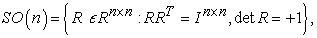(1)

where In×n is n x n the identity matrix. For n = 2, SO(2) group has the following form: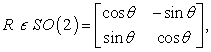(2)

where θ is the magnitude of rotation. These matrices are smooth, cyclic and unique with respect to theta and thus correspond to points on S1 (i.e. their spaces are diffeomorphic). The actions of two Lie groups are also compatible with the matrix product of two SO(2) elements, that is equivalent to the modular sum of the corresponding S1 elements. This compatibility means that S1 and SO(2) are fully isomorphic to each other, but this is not a general requirement for groups sharing a manifold structure. In the same way the circle generalizes to higher dimensions either via the direct product as a torus or as a higher-dimensional sphere, direct products of multiple (S1, +mod k) groups produce toroidal Lie groups and extensions of SO(n) produce rotations through higher-dimensional spaces. Between Sn-1 and SO(n)there is not the direct relation. For example, S2 does not admit any Lie group structure and SO(3) corresponds to the set of oriented positions on the sphere .

3.1. The Special Euclidean Group

In the case of rigid bodies moving in the plane, for which the frame is defined by its two-dimensional position and one component of orientation, it might be natural to take these components independently and use the configuration group(R2 × S1, +), because the addition of translations and changes in orientation is easier than the matrix product.

Further, instead it we will use the special Euclidean group (SE(2), ×), which better captures the relative motion of rigid bodies than group (R2× S1, +). The set on which this group is defined is given by: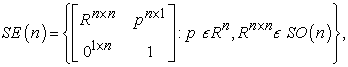(3)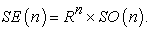(4)

For n = 2, an element g ϵ SE(2) has components (x, y, θ) and is typically represented as a homogeneous matrix: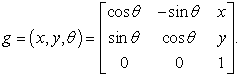(5)

This structure, containing an SO(2) element for orientation and an R2 element for position, represents the semi-direct product SO(2) R2, with rotations acting on each other and on the translational components, but the translational components only acting on each other. Geometric meaning of this special Euclidean group is that, the rotation is given by matrix SO(2) and the displacement is given by vector t: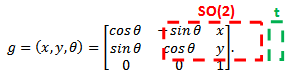(6)

Elements of SE(2)have four common interpretations for planar systems:

1. the position and orientation of rigid bodies

2. the position and orientation of coordinate frames

3. actions that move a rigid body or coordinate frame with respect to a fixed coordinate frame

4. actions that take a point in one coordinate frame, and find the equivalent point in a second coordinate frame

As discussed above, the first and second interpretations are closely linked—the position and orientation of a rigid body identifies a body coordinate frame aligned with the object’s longitudinal and lateral axes and vice versa. The third and fourth interpretations relate to SE(2)being not just a space but a Lie group, making every configuration also an action .

### 4. The Fiber Bundle

We will assume that the position variables are elements of a set that has a group structure such as Rn, SO(n) or SE(n). Additionally, the position variables belong to the configuration manifold and hence the position variables are governed by a Lie group structure. For mechanical system, we assume that a general configuration manifold is usually denoted by Q = G × M, where G is the fiber space specifying the position of the robot and M is the base space specifying the internal shape of the robot.

For a manifold Q with a base subspace M and a projection map π : Q → M, we define a fiber G(r) as the pre-image of r ϵ M in Q with respect to the map π, that is, G = π1(r). Then Q is the fiber bundle if for every neighborhood U M of r we have π-1(U) is homeomorphic to G × U, that is, locally we have Q M × G.

If the fiber G has a group structure then Q is a principal fiber bundle and if Q = G × M globally, then Q is a trivial fiber bundle. The configuration space of all mechanical systems is the trivial principal fiber bundle .

We will show an example of the configuration space on the Snakeboard. It is composed of one rigid link, a rotor pivoting around the center of the link and two passive wheel sets pivoting at the each of the distal ends of the link. The no sideways slippage of these two sets of wheels provides the two non-holonomic constraints. We attach a body coordinate frame to the middle of the center link as shown in the following figure Figure 2. The position and orientation of the body frame is represented by the fiber variables (x, y, θ), while the two internal degrees of freedom representing the wheel axes and rotor angles are denoted by the base variables 1, α2). The configuration space of this Snakeboard is: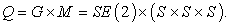(7)

### 5. The Actions and Lifted Actions

5.1. The Left and Right Action on the Special Euclidean Group

In general, the groups may have the left action Lhg = h ○ g and the right action Rhg = g ○ h, where an element h acts on an element g. At Abelian groups apply that the left and right action are identical Lhg = Rhg. The special Euclidean group SE(2) is not commutative and therefore these actions are not equivalent.

Let group elements g, h ϵ SE(2), then the initial configuration of rigid body with respect to the inertial frame has the matrix form:(8)

If a group element h has coordinates: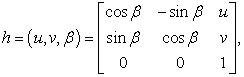(9)

then the left action of group element h on group element g is equal: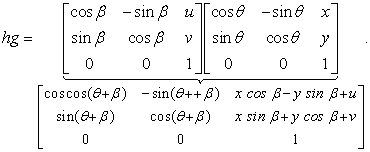(10)

The resulting matrix physically represents a new configuration of the rigid body, which is given by the vector: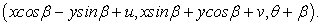(11)

This left action transforms g first by rotating of body around the origin about the angle β and then translates it about u and v, as is shown in the following figure [2, 5].

The right action of group element h on group element g has form: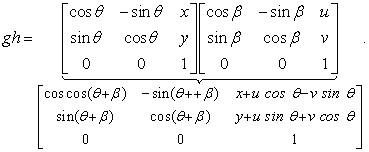(12)

Again, the new configuration of rigid body is given by the vector: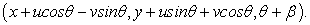(13)

In this case, the right action moves body from the initial position g about value h with respect to the initial position (x, y, θ).

5.2. The Lifted Actions

When analyzing the kinematics and dynamics of the system, it is generally important to consider not only about its configuration q, but also the velocity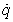with which its configuration changes. Together, these quantities create the state of system. Velocity vectors are represented as elements of the tangent space TqQ associated with the configuration space Q of the system. The tangent space to a manifold can be viewed as alinearization of the manifoldor the vector space that the best approximates the manifold in given point and can only exist if the manifold is at least differentiable in one point. The intuition definition of the tangent space is shown in Figure 6 (on the left), where the velocity of a point moving along the circle, can always be represented as a vector lying in the line tangent to the circle at its current location, with magnitude corresponding to the velocity of point. The tangent space to points moving along line (Figure 6-on the right) is a line. At higher-dimensional surfaces, such as the sphere, the tangent space is two-dimensional plane touching the sphere. In general, the tangent spaces to n-dimensional manifolds are Rn vector spaces. The set of all the tangent spaces to the manifold creates the tangent bundle TQ .

Individual tangent spaces in a tangent bundle are independent from one another, so in the general case there is no well-defined method for comparing or adding vectors in different spaces. When transformations are defined between configurations, as is the case for Lie groups, these transformations may have associated lifted actions that map between equivalent vectors in separate tangent spaces and allow them to be compared. Specifically, on Lie groups a left action Lh applied to an initial configuration g is accompanied by a left lifted action TgLh that takes vectors from the starting tangent space TgG and finds their equivalent vectors in the ending tangent space ThgG: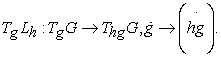(14)

A system’s body velocity ξ is its velocity expressed in the instantaneous local coordinate frame (Figure 7). For a planar system, the components of ξ can be characterized as the forward velocity ξx, lateral velocity ξy and rotational velocity ξθ. The body velocity ξ plays an important role in kinematic and dynamic analysis, where forces and constraints are often defined in terms of body coordinates.

At a strictly mechanical level, the body velocity of a rigid body with orientation θ can be calculated from the world velocity g by rotating the translational component by-θ and leaving the rotational component unchanged. Then the body velocity has form :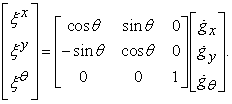(15)

Similarly, the world velocity can be calculated from the body velocity by inverting previous relation (Figure 8):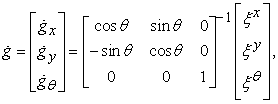(16)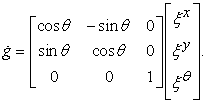(17)

For many purposes, these relations are all that is needed to make effective use of the body velocity. However, by examining their roots in the structure of SE(2), specifically the left lifted action, we can gaingreater insight into rigid body motion and apply some powerful mathematical tools to these systems.

Let g, h ϵ SE(2), where g = (x, y, θ) and h = (u, v, β). The left lifted action on SE(2)is the differential of left action: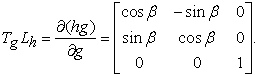(18)

The left lifted action has some especially interesting properties when h is set equal to g-1. The combination of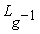and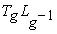take a rigid body from g and place it at the origin with equivalent body velocity (Figure 9):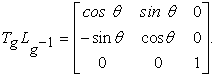(19)

After substituting relation (19) into equations (15) and (16), we can express the body velocity and the world velocity by using the following equations: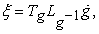(20)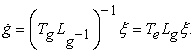(21)

The right lifted action on SE(2) for g = (x, y, θ) and h = (u, v, β) is calculated as the differential of right action: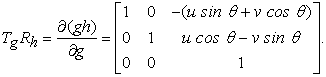(22)

The right lifted action preserves the spatial velocity, i.e. the velocity of the imaginary point on rigid body that is instantaneously over the origin and is calculated as (Figure 10):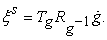(23)

By inverting of relation (23), the spatial velocity of rigid body maps to the world velocity of any chosen body frame of rigid body: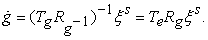(24)

Using adjoint operators we can transform the body velocity ξ on the spatial velocity ξs and vice versa. These operators we get by pairing of lifted actions and together they are able to realize the adjoint actions of Lie groups. Adjoint action Adg on SE(2) maps the body velocity ξ to the spatial velocity ξs. The following relation represents the dependent between ξs and ξ :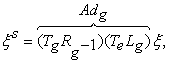(25)

and substituting for lifted actions we get form: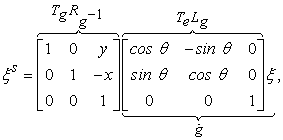(26)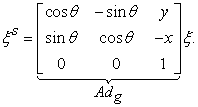(27)
Figure 11. Examples of velocities, lifted actions and adjoint action on SE(2) group 

By inverting of the adjoint action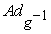we obtain the relation of depending ξ on ξs: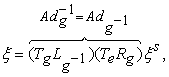(28)

and again substituting for lifted actions the body velocity ξ has form: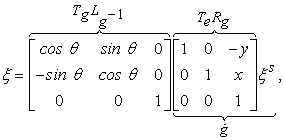(29)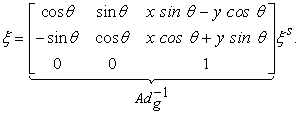(30)

### 6. Conclusion

This paper contains the theoretical background of geometric mechanics and differential geometry. It includes the basic building blocks and their configuration manifolds for describing of mechanical systems and here were mentioned basic relations to calculate actions between groups, velocities, lifted and adjoint actions.

### Acknowledgement

The authors would like to thank to Slovak Grant Agency – project VEGA 1/1205/12 "Numerical modelling of mechatronic systems". This contribution is also the result of the grant project: Tatra Bank – TRANSBRIDGE "Innovative methods for designing of robotic mechanisms".

### References

  Elie A. Shammas, Generalized Motion Planning for Underactuated Mechanical Systems, The dissertation thesis,Carnegie Mellon University, Pittsburgh, March 20, 2006.In article  Ross L. Hatton, Howie Choset, An Introduction to Geometric Mechanics and Differential Geometry, December 6, 2011.In article  James Patrick Ostrowski, The Mechanics and Control of Undulatory Robotic Locomotion, The dissertation thesis, California Institute of Technology Pasadena, September 19, 1995.In article  Silvia Ebetiuc, Staab Haraldw, “Applying Differential Geometry to Kinematic Modeling in mobile Robotics”, 2005 WSEAS Int. Conf. on Dynamical systems and control, Italy, November 2-4, 2005,106-112.In article  Ross L. Hatton, Howie Choset, “Connection Vector Fields for Underactuated Systems”, Proceedings of the 2nd Biennial IEEE/RAS-EMBS International Conference on Biomedical Robotics and Biomechatronics Scottsdale, USA, October 19-22, 2008, 451-456.In article  Elie Shammas, Klaus Achmidt, Howie Choset, “Natural Gait Generation Techniques for Multi-bodied Isolated Mechanical Systems”, Proceedings of the 2005 IEEE International Conference on Robotics and Automation Barcelona, Spain, April 2005, 3664-3669.In article CrossRef  Ross L. Hatton, Howie Choset, “Optimizing Coordinate Choice for Locomoting Systems”, 2010 IEEE International Conference on Robotics and Automation Anchorage Convention District, USA, May 3-8, 2010, 4493-4498.In article